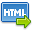Volume No. :   5

Issue No. :  1

Year :  2013

ISSN Print :  0975-4393

ISSN Online :  2349-2988Registration

## Basic concept of Linear Algebraic groups and Lie algebra

 Address: Shivani Sharma1* and R P Sharma2 1Assistant Professor, Sri Sai University, Palampur, HP -176102 2Professor, Department of Mathematics, Himachal Pradesh University, Shimla –171005 *Corresponding Author
DOI No:

ABSTRACT:
In view of close association between the general linear algebra and general linear groups, it is natural to study the concepts of classical linear groups before Lie algebras and we study these groups and Engel’s Theorem. In this paper, we study some basic results, which are useful to provide concise information for the subsequent sections. Here, we study linear algebraic groups and the bilinear forms on the space of column matrices, which are useful to prove the further results. The study of Lie Algebras was motivated by the vector space of Linear Transformation (End (V)) endowed with a new operation known as bracket operation or commutator: [xy] = xy–yx, the operation on right hand side are the usual compositions of linear transformations. The bracket operation is in general neither commutative nor associative. This particular Lie Algebra with this new algebraic structure is denoted by gl(v) and is called as General Linear Algebra. Besides being useful in many parts of mathematics and physics, the theory of Lie Algebras is attractive. The theory of Lie Algebras constitutes a good foundation to representation theory. Many results, e.g. Conjugancy Theorems can be proved by using elementary Lie Algebra methods, avoiding the use of algebraic geometry. The classification of finite dimensional complex semisimple Lie algebra is one of the most beautiful theories in mathematics. The purpose of this paper is to give a small sketch of a part of this theory up to Engel’s Theorem which is important to study a very valuable tool “ Jorden chevelly decomposition” in the representation of Lie algebras. Apart from the basic concepts like ideals, homomorphism, automorphism, solvability and nilpotency; we study one substantial theorem (Engel’s Theorem) in this Paper.
KEYWORDS:
Linear algebraic group , Engle’s theorem.
Cite:
Shivani Sharma, R P Sharma. Basic concept of Linear Algebraic groups and Lie algebra. Research J. Science and Tech 5(1): Jan.-Mar.2013 page 20-24.[View HTML][View PDF]

Visitor's No. :   205771